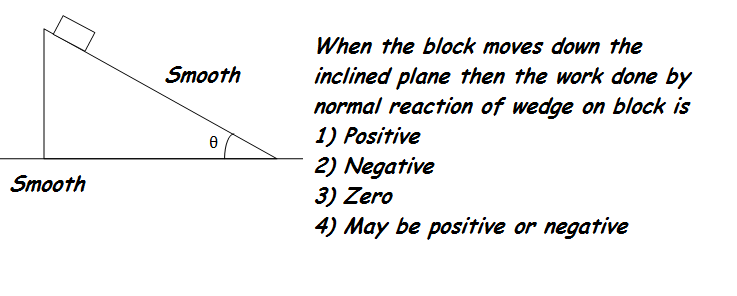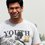# Thought Of The Day_4_Work N Frames!!

$Comments Invited!!$Note by Rohit Gupta
6 years, 2 months ago

This discussion board is a place to discuss our Daily Challenges and the math and science related to those challenges. Explanations are more than just a solution — they should explain the steps and thinking strategies that you used to obtain the solution. Comments should further the discussion of math and science.

When posting on Brilliant:

• Use the emojis to react to an explanation, whether you're congratulating a job well done , or just really confused .
• Ask specific questions about the challenge or the steps in somebody's explanation. Well-posed questions can add a lot to the discussion, but posting "I don't understand!" doesn't help anyone.
• Try to contribute something new to the discussion, whether it is an extension, generalization or other idea related to the challenge.

MarkdownAppears as
*italics* or _italics_ italics
**bold** or __bold__ bold
- bulleted- list
• bulleted
• list
1. numbered2. list
1. numbered
2. list
Note: you must add a full line of space before and after lists for them to show up correctly
paragraph 1paragraph 2

paragraph 1

paragraph 2

[example link](https://brilliant.org)example link
> This is a quote
This is a quote
    # I indented these lines
# 4 spaces, and now they show
# up as a code block.

print "hello world"
# I indented these lines
# 4 spaces, and now they show
# up as a code block.

print "hello world"
MathAppears as
Remember to wrap math in $$ ... $$ or $ ... $ to ensure proper formatting.
2 \times 3 $2 \times 3$
2^{34} $2^{34}$
a_{i-1} $a_{i-1}$
\frac{2}{3} $\frac{2}{3}$
\sqrt{2} $\sqrt{2}$
\sum_{i=1}^3 $\sum_{i=1}^3$
\sin \theta $\sin \theta$
\boxed{123} $\boxed{123}$

Sort by:

Since normal( Between block and wedge) is not a dissipative force hence net work done by it on the system is zero.

Now the lower wedge is getting velocity because of the normal force hence it is doing positive work on the wedge , since net work is zero it implies normal force does negative work on the block.

Ans) Negative

Another way to analyse the FBD of the block

The normal force is making an angle \theta with the positive y-axis and the block's displacement has two components one perpendicular to block and one along the -x axis. So work done in first component is zero and work done in second component is -Nxsin(\theta) ( The angle between normal and displacement(x) is 90+\theta)

It is clear work done is negative.

- 6 years, 2 months ago

Bhiya Can U give me ur Email ID ?

- 6 years, 2 months ago

Best of luck Bhaiya for Sunday. I am sure U will get AIR 1. $\ddot\smile$

- 6 years, 2 months ago

If by chance I would get a rank just under 100 I would be over the moon and you are talking about 1 Ha Ha Ha.

- 6 years, 2 months ago

Yes You are Right Here..!! Kudos..!!

- 6 years, 2 months ago

W=F.S when force is constant.
As clearly seen from diagram,angle b/w force and displacement is 90.
Hence, work done is 0

- 6 years ago

@Akhil Bansal I am sorry but the angle between force and displacement here is not 90..!!

- 6 years ago

Can you see this ?

- 6 years ago